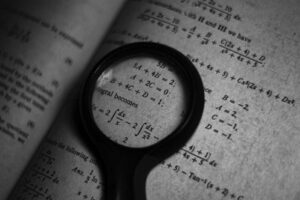# Square Root and Cube Root Questions Answers Quantitative Aptitude

Looking for Square Root and Cube Root Model Paper Pdf then you are on the right page. Here candidates can find the study material & model paper for the competitive examination.Hence, click on the respective link in the table below and start downloading the Square Root and Cube Root Question Paper Pdf for free. We have given the free Square Root and Cube Root exam model question paper with answers to help the candidates. Hence, go through the article and find the respective Square Root and Cube Root Sample Paper and start your preparation.

Here are links for respective Square Root and Cube Root Questions in Quantitative Aptitude. Aspirants who are going to appear for various competitive exams are advised to check the Square Root and Cube Root Questions pattern before starting preparation. Knowledge of Square Root and Cube Root helps the applicants a lot in the preparation.

All questions relevant to Square Root and Cube Root are collected from previous year question papers form Bank, IBPS, SSC, PSC, CAT, MAT and other competitive exam.

## Model Questions on Square Root and Cube Root

1. If { \left( \frac { 3 }{ 4 } \right) }^{ 3 }{ \left( \frac { 4 }{ 3 } \right) }^{ -7 }={ \left( \frac { 3 }{ 4 } \right) }^{ 2x }, then x is:

1. 2.5
2. -2
3. 2
4. 5

2. Number of digits in the square root of 62478076 is:

1. 3
2. 4
3. 5
4. 6

3. A rational Number between \frac {3}{4} and \frac {3}{8} is

1. \frac {16}{9}
2. \frac {9}{16}
3. \frac {12}{7}
4. \frac {7}{3}

4. Find the simplest value of 2\sqrt {50}+\sqrt {18}\sqrt {72} (Given \sqrt {2}=1.414)

1. 10.312
2. 8.484
3. 4.242
4. 9.898

5. The numerator of a fraction is 4 less than its denominator. If the numerator is decreased by 2 and the denominator is increased by 1, then the denominator becomes eight times the numerator. Find the fraction

1. \frac {4}{8}
2. \frac {2}{7}
3. \frac {3}{8}
4. \frac {3}{7}

6. If {x}^{2}=y+z, {y}^{2}={z}^{x} and {z}^{2}=x+y, then the value of \frac {1}{1+x}+\frac {1}{1+y}+\frac {1}{1+z} is

1. 2
2. 0
3. -1
4. 1

7. If a=2, b=3 then { \left( { a }^{ b }+{ b }^{ a } \right) }^{ -1 } is

1. \frac {1}{31}
2. \frac {1}{17}
3. \frac {1}{21}
4. \frac {1}{13}

8. The smallest positive integer which when multiplied by 392, gives a perfect square is

1. 2
2. 3
3. 5
4. 7

9. The forth root of 24010000 is

1. 7
2. 49
3. 490
4. 70

10. The greatest 4 digit member which is perfect square is

1. 9999
2. 9909
3. 9801
4. 9081

11. The value of \frac { 4+3\sqrt { 3 } }{ 7+4\sqrt { 3 } } is

1. 5\sqrt {3}-8
2. 5\sqrt {3}+8
3. 8\sqrt {3}+5
4. 8\sqrt {3}-5

12. Which of the following is the minimum value of the sum of two integers whose product is 24?

1. 25
2. 11
3. 8
4. 10

13. If { ({ 2 }^{ 3 }) }^{ 2 }={4}^{x} then {3}^{x} is equal to

1. 3
2. 6
3. 9
4. 27

14. Evaluate \frac { \sqrt { 24 } +\sqrt { 6 } }{ \sqrt { 24 } -\sqrt { 6 } }

1. 2
2. 3
3. 4
4. 5

15. A farmer divided his herd of n cows among his four sons, so that the first son gets one-half the herd, the second one-fourth and the third son one-fifth. If fourth son get 7 cows, then the value of n is

1. 240
2. 100
3. 180
4. 140

## Read More Questions on Aptitude

16. By what least number should 675 be multiplied to obtain a number which is a perfect cube?

1. 7
2. 8
3. 5
4. 6

17. If 21 is added to a number it becomes 7 less than thrice of the number. Then the number is

1. 14
2. 16
3. 18
4. 19

18. If the sum of two numbers be multiplied by each number separately, the products so obtained are 247 and 114. The sum of the number is

1. 19
2. 20
3. 21
4. 23

19. Find a number one seventh of which exceeds its eleventh part by 100

1. 1925
2. 1825
3. 1540
4. 1340

20. A student was asked to divide a number by 6 and add 12 to the quotient. He, however first added 12 to the number and then divided it by 6, getting 112 as the answer. The correct answer should have been

1. 124
2. 122
3. 118
4. 114